Balancing Car - Part 1

The main reference of this project is this tutorial.

The bipolar stepper motor we are going to use in this lab was ordered from here:

https://www.omc-stepperonline.com/nema-17-bipolar-59ncm-84oz-in-2a-42x48mm-4-wires-w-1m-cable-and-connector.html

Watch: How a stepper motor works?

1. Basics
1.1. Drive the motor using the L298N H-Bridge driver

The first step is to use the basic circuit and code to test drive the stepper motor.
What you need:
1. A power supply can provide 12 V DC and 2 A as the minimum.
2. A NEMA17 stepper motor
3. One Arduino UNO board
4. An L298N stepper motor driver module
5. A breadboard and jumping wires.

To make the following connection:The real circuit looks like this: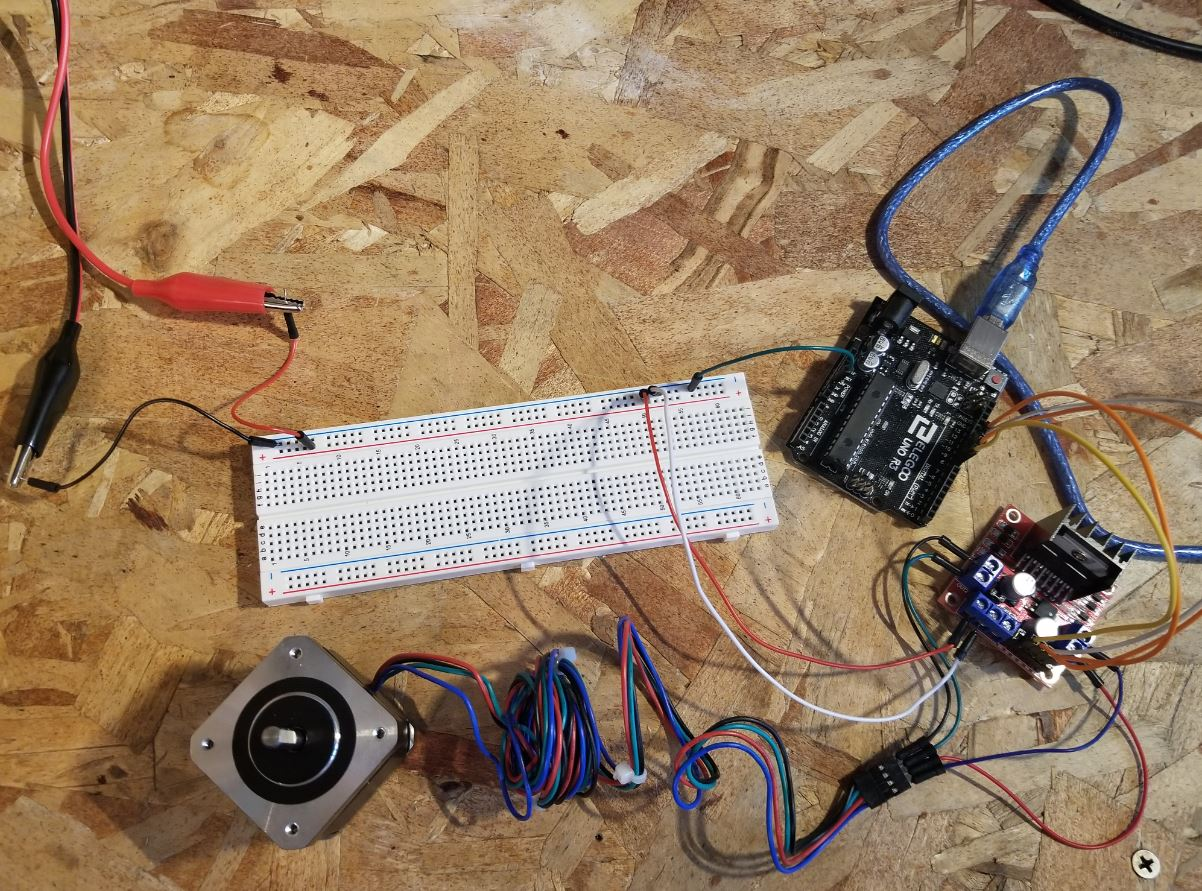The Arduino code to kick if off: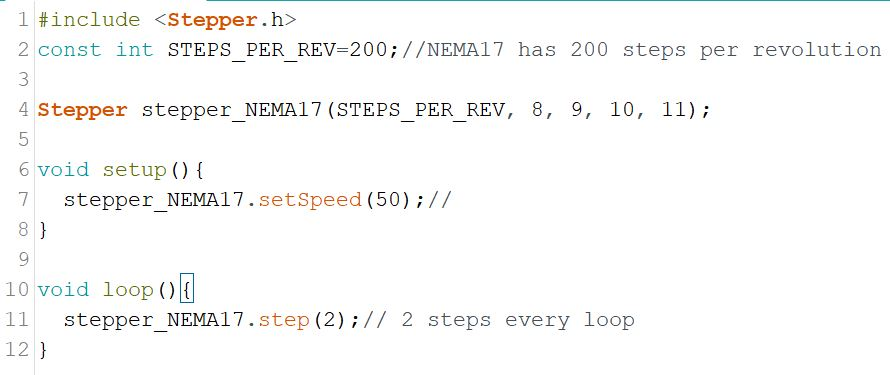In NEMA17's datasheet, the STEP ANGLE parameter tells you the 'steps per revolution' you have to define in your sketch. Every step moves 1.8 degree, there are 360 degrees per revolution so totally 200 steps.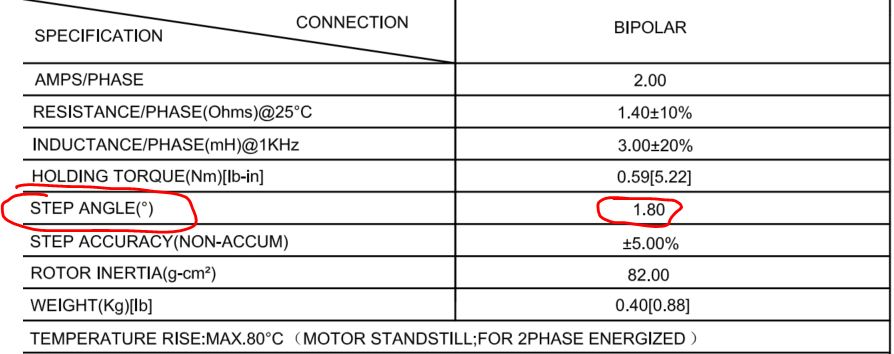The demonstration video:

Task 1: Repeat the work demonstrated in the video. (CE432 students, please skip Task 1 in this tutorial)

1.2 Use a potentiometer to change the speed in realtime

You can use a potentiometer to control the RPM of the stepper motor.
Make the following connections:The sketch.

The demonstration video:

Task 2: Repeat the work demonstrated in the video. (CE432 students, please skip Task 2 in this tutorial)

1.3 Use the A4988 stepper motor driverA4988 is an alternative product to the L298N driver.

The A4988 is a microstepping driver for controlling bipolar stepper motors which has built-in translator for easy operation. This means that we can control the stepper motor with just 2 pins from our controller, or one for controlling the rotation direction and the other for controlling the steps.

The Driver provides five different step resolutions: full-step, haft-step, quarter-step, eight-step and sixteenth-step. Also, it has a potentiometer for adjusting the current output, over-temperature thermal shutdown and crossover-current protection. Its logic voltage is from 3 to 5.5 V and the maximum current per phase is 2A if good addition cooling is provided or 1A continuous current per phase without heat sink or cooling.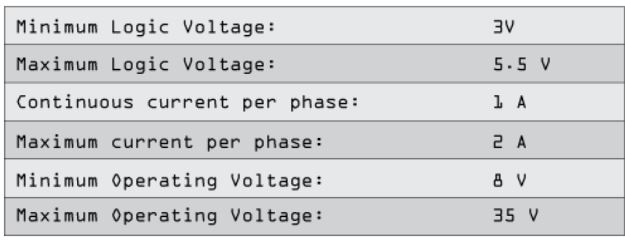The next two 2 pins, Step and Direction are the pins that we actually use for controlling the motor movements. The Direction pin controls the rotation direction of the motor and we need to connect it to one of the digital pins on our microcontroller.
With the Step pin we control the mirosteps of the motor and with each pulse sent to this pin the motor moves one step. So that means that we don’t need any complex programming, phase sequence tables, frequency control lines and so on, because the built-in translator of the A4988 Driver takes care of everything. Here we also need to mention that these 2 pins are not pulled to any voltage internally, so we should not leave them floating in our program.

Next is the SLEEP Pin and a logic low puts the board in sleep mode for minimizing power consumption when the motor is not in use. Next, the RESET pin sets the translator to a predefined Home state. This Home state or Home Microstep Position can be seen from these Figures from the A4988 Datasheet. So these are the initial positions from where the motor starts and they are different depending on the microstep resolution. If the input state to this pin is a logic low all the STEP inputs will be ignored. The Reset pin is a floating pin so if we don’t have intention of controlling it with in our program we need to connect it to the SLEEP pin in order to bring it high and enable the board.The next 3 pins (MS1, MS2 and MS3) are for selecting one of the five step resolutions according to the above truth table. These pins have internal pull-down resistors so if we leave them disconnected, the board will operate in full step mode. The last one, the ENABLE pin is used for turning on or off the FET outputs. So a logic high will keep the outputs disabled.

Datasheet of the A4988 driver IC can be downloaded here. The module's pinout and specs can be found here.

Some notes:
- Every pulse will create one step, which is 1.8 degree (if it is a fll step, MS1 MS2 MS3 is  0 0 0), the pulse width cannot be too small. The example uses 500 us as the pulse width and it works fine. I tried 400 us and it doesn't work. You can also use wider pulses, say 1000 us, the rotation speed will be slower.
- 7.4 V for VMOT also works which means you can use a 2-cell lipo battery.
- The 47 - 100 uF cap shorting VMOT to GND can be absent without causing anything, but it is highly recommended to have it in place.

The hardware connection: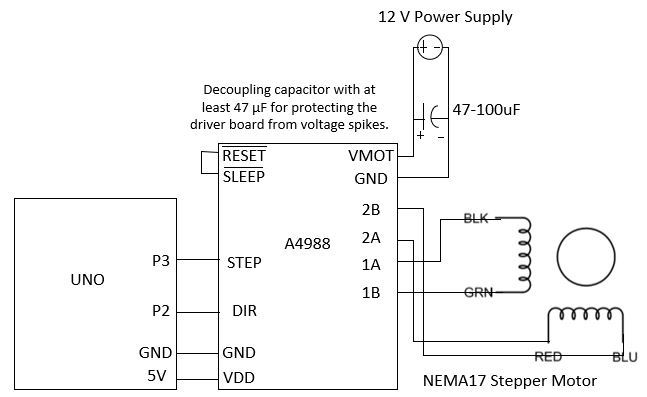The sketch.

The demonstration video:

Task 3: Repeat the work demonstrated in the video. (CE432 students, please skip Task 3 in this tutorial)

1.4 Use the A4988 driver and a potentiometer to adjust the motor speed in realtime.

Follow the instructions in section 1.2 and try to repeat the same results using the A4988 driver.

The demonstration video:

Task 4: Repeat the work demonstrated in the video. (CE432 students, please skip Task 4 in this tutorial)

2. Test the MPU6050 accelerometer/gyroscope sensor
2.1 Understand acceleration and angular velocity

The pinout of MPU-6050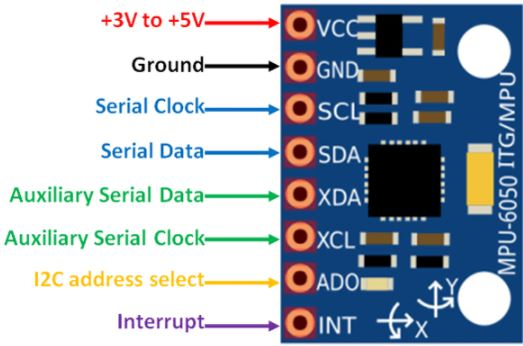The schematic of the module: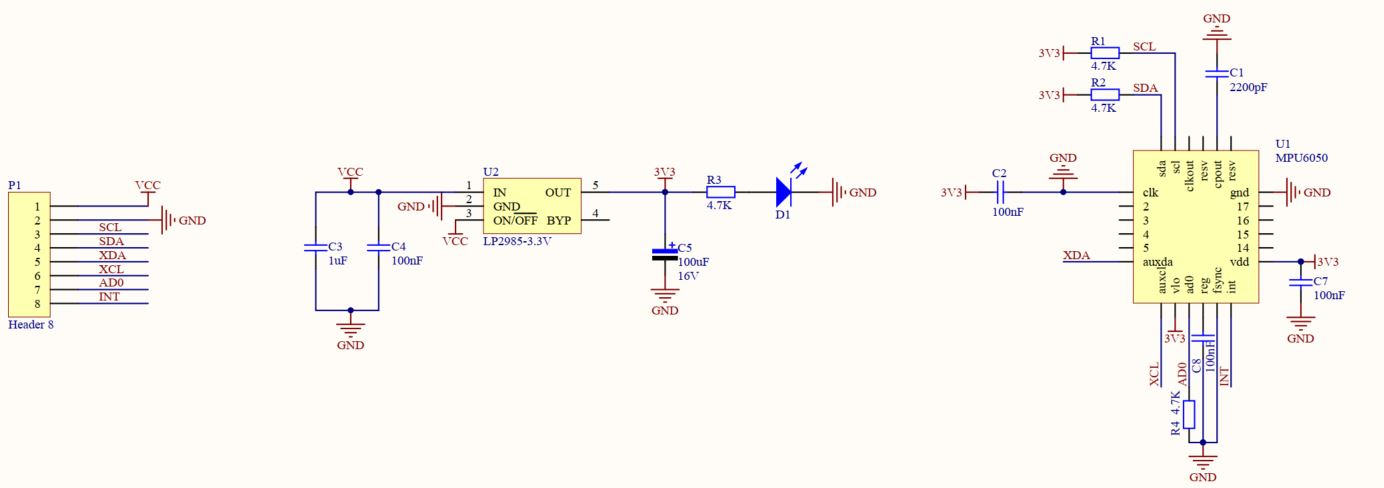Table of voltage ratings: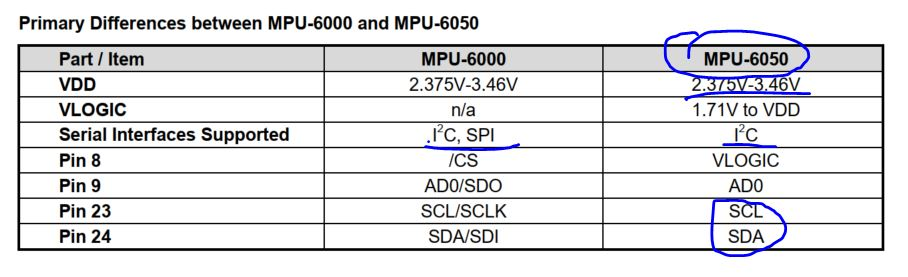MPU-6050 Axis: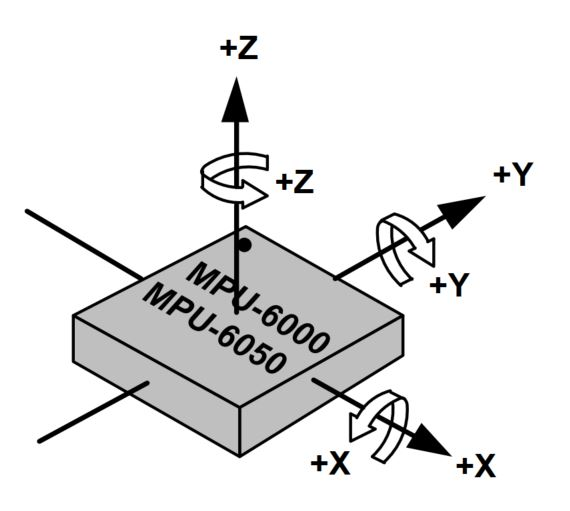MPU I2C communication:The key registers: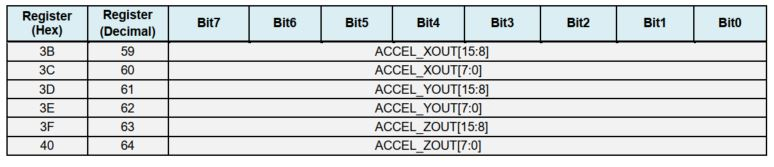Binary readout data / LSB Sensitivity = xxx LSB / (LSB / g) = xxx g.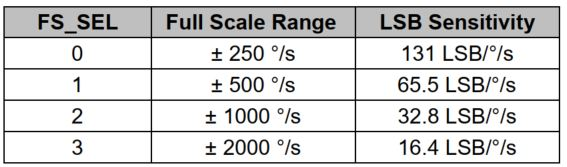Binary readout data / LSB Sensitivity = xxx LSB / (LSB / (deg/s)) = xxx deg/s. This is the angular velocity but how do you get the rotated angles in realtime? - You must know the time spent for each 'void loop ()' function.

Use the following software and hardware to test your accelerometer and gyroscope before it's being connected to the car.

From the UNO's pinout, you can idenfy the I2C pins are A4 and A5:Make the following hardware connections: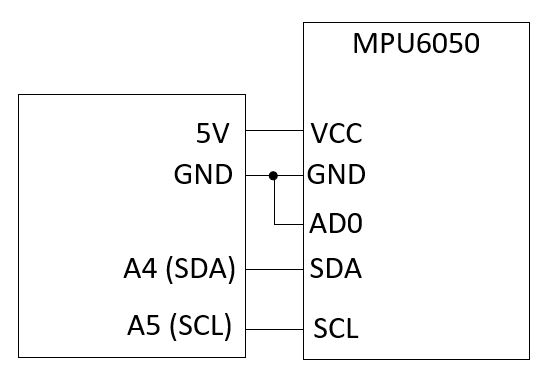Use this example to test your acceleration and gyroscope readings respectively. Check out MPU6050's datasheet and register map if you have questions about the example sketch.

By holding the MPU6050 sensor at different gestures, you can understand what the accelerometer and the gyroscope are measuring.

The Gyro measures angular velocity. Therea are three axes X, Y, and Z, the direction of them are indicated on the module's PCB board.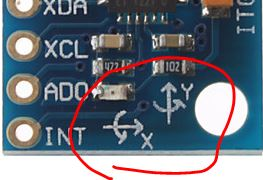The Z axis in the snippet above points out of your monitor and perpendicular to the PCB board. Let's take the airplane's X, Y, and Z axes as the example.

For airplane control, Pitch, Roll and Yaw are defined as the rotation around X, Y and Z axis. Below as a picture to illustrate the definition.If the MPU is idle, no movement and no rotation, the Gyro readout should be all zeros.

Look at the following demonstration that I held the MPU module at different angles statically, the readout is always zero.However, the acceleration readout values reacts to the angular changes. (I have converted the unit of the readings to 'g' for the following tests).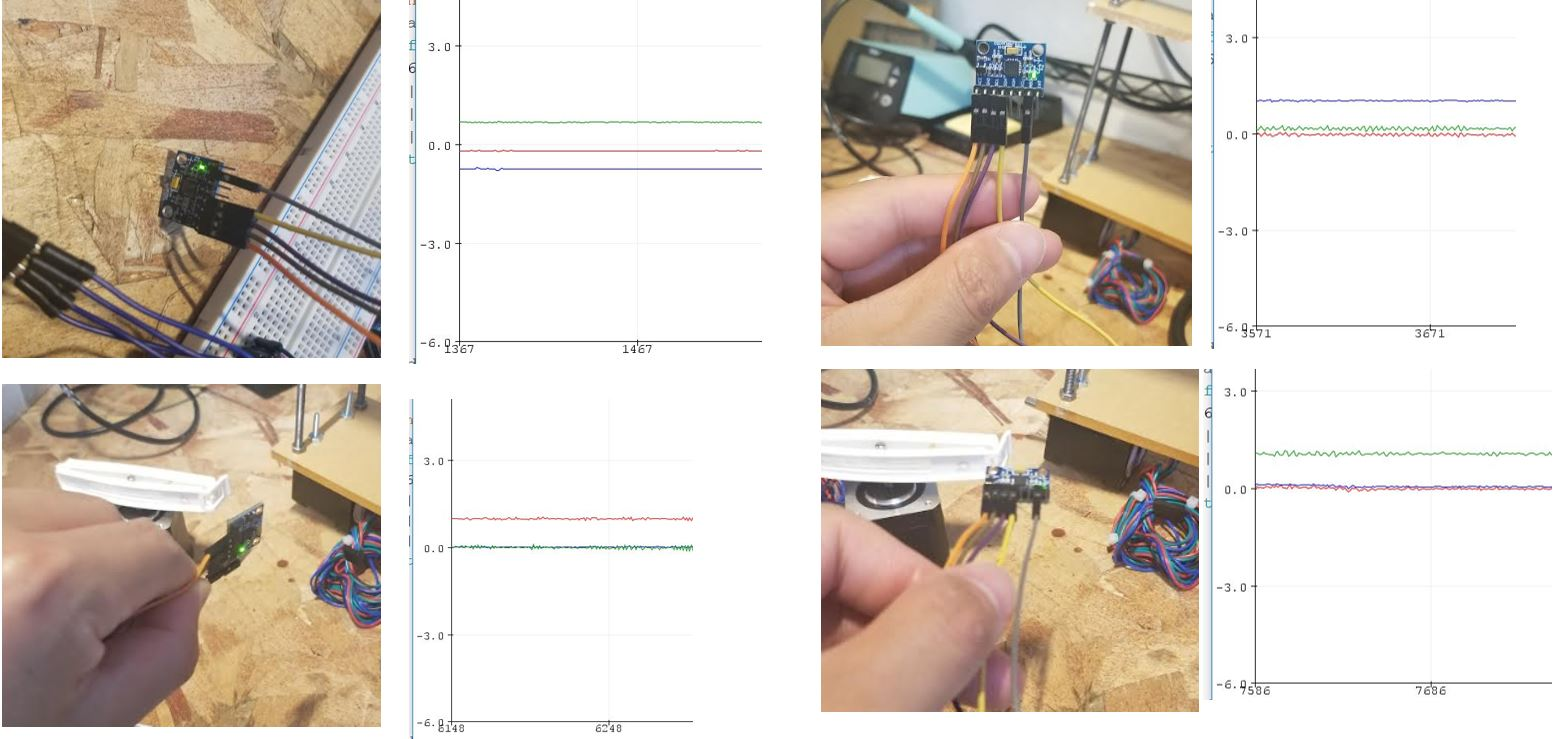Keep in mind that gravity always exists so if you plug your MPU module on a breadboard vertically as follows: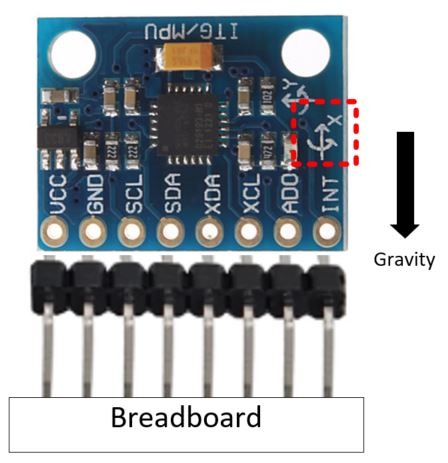You are supposed to receive something close to 8192 for Accel_X.

The number '8192' comes from the following configuration according to the register map.Task 5: Modify the MPU's code I provided in the last section to display the raw acceleration data of the X axis as shown in the following figure. The MPU module is placed perpendicular to the board.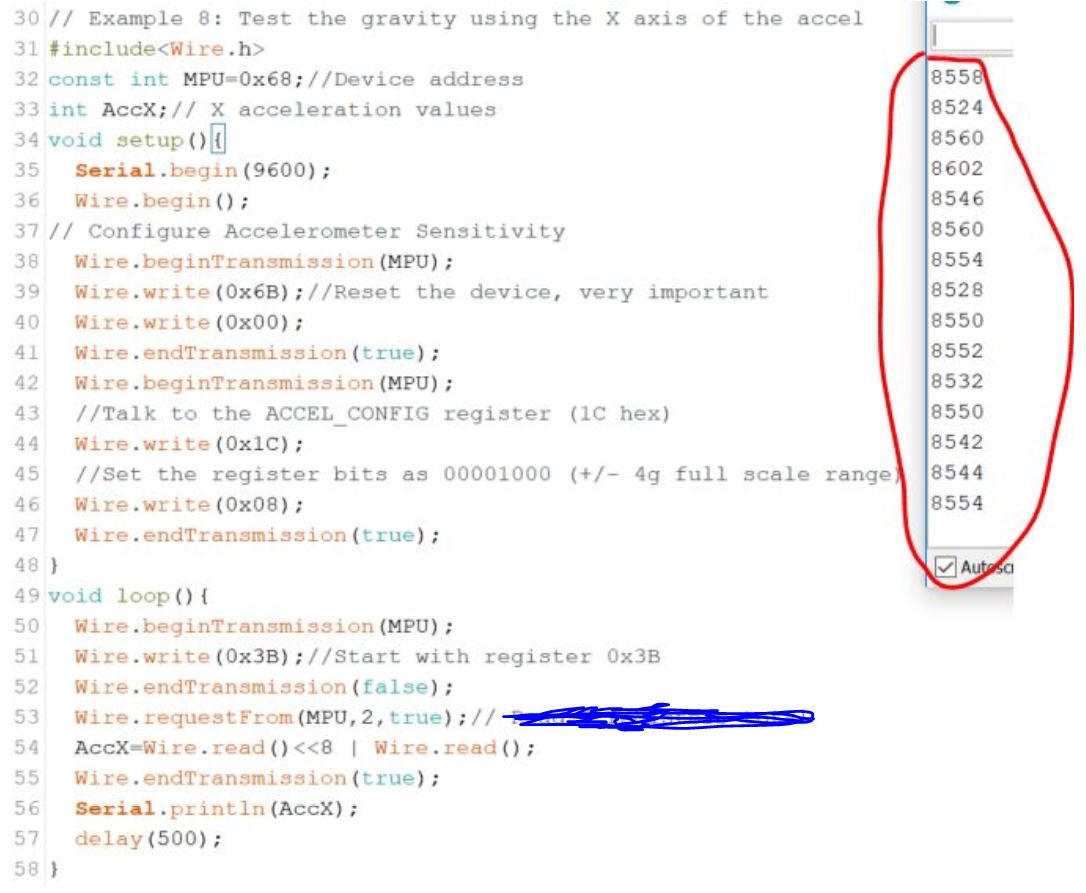(All these 8500+ values are supposed to be 8192 but you can tell the level of the deviations by holding it by hand and the system errors of itself.
The values are positive so disregard the direction of the arrow printed on the MPU module for the X axis. )

The code starts with the 0x3B register for data reading because the 2-byte X axis data is stored in 0x3B and 0x3C.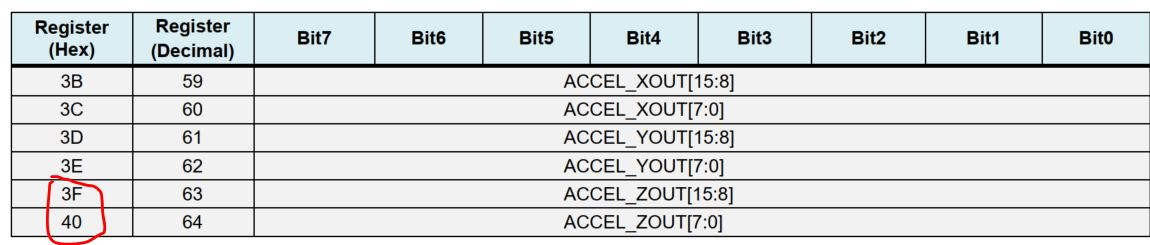2.2 Remove the offset of the Gyro's readings

In last section you may have noticed that Gyro's readings are not perfectly 0:Here is the piece of the code to peform the calculation.

if(receive_counter % 15 == 0) digitalWrite(13, !digitalRead(13));        //Change the state of the LED every 15 loops to make the LED blink fast
Wire.beginTransmission(MPU);                                                            //Start communication with the gyro
Wire.write(0x45);                                                                 //Gyro Y_OUT
Wire.endTransmission();                                                 //End the transmission
Wire.requestFrom(MPU, 2);                                               //Request 2 bytes from the gyro
delayMicroseconds(3700);                                                //Wait for 3700 microseconds to simulate the main program loop time, CRITICAL!!
}
gyro_pitch_calibration_value /= 500;
}

Task 6: Use the code above to report the averaged 'gyro_pitch_calibration_value' to your serial monitor (with 500 ms delays in between the readings).

2.3 The loop_timer variable

There is a time delay created by the feedback loop of the PID controller. In this experiment, the time delay is caused by the finite oscillation frequency of the crystal and the time constants of the entire circuit.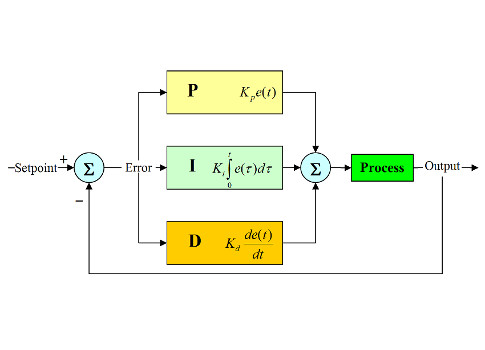To unify the 'delta_t' of the PID controller of every loop of the loop() fuction, you can start timing the loop time by adding the following line in the end of the setup() function.

setup(){
.......................
.......................
loop_timer = micros() + 4000;                                             //Set the loop_timer variable at the next end loop time
}

The micros() function returns the number of microseconds since the Arduino board began running the current program. This number will overflow (go back to zero), after approximately 70 minutes. On 16 MHz Arduino boards (e.g. Duemilanove and Nano), this function has a resolution of four microseconds (i.e. the value returned is always a multiple of four).

This is to add the time spent on the setup() function to 4000 us (4 ms). You need it to be added to 4000 us since 4000 us is the desired loop time (delta_t) for the PID controller. Of course 4000 us is not the only value that you can use here. This is value is identified by testing the time delay of evey loop and picking up a value that is a little larger than that time.

Add micros() to 4000 is to set the standard time delay of the loop() function to 4000 us. If the loop() function spends less it should be manually delayed inside the function until it uses all 4000 us. To do this, in the end of the loop() function, add the following line:

loop(){
.......................
.......................
while(micros() < loop_timer); // while micros() < loop_timer, hold there and wait
loop_timer += 4000;           // once micros() > loop_timer, add 4000 us to loop_timer.

}

The setup() function only runs once, so the time spent on it is not counted in 'delta_t'.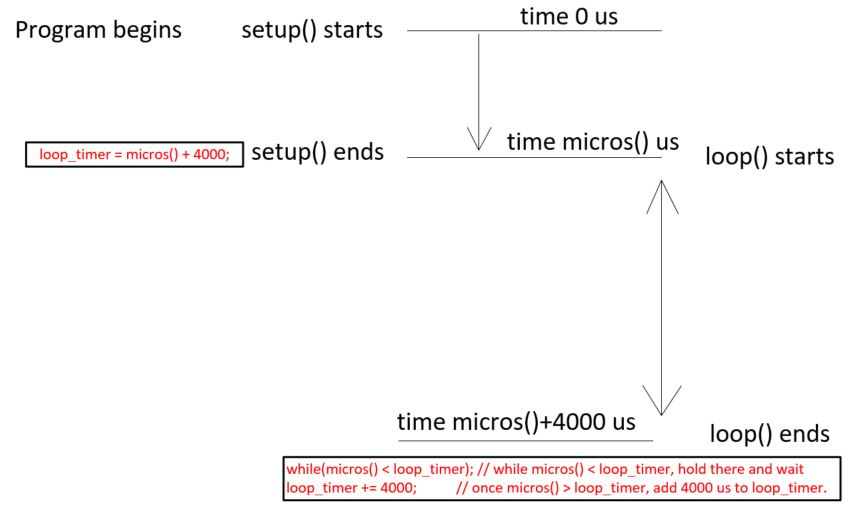Task 7: Use this technique to blink an LED at 1 Hz (the program shouldn't have anything irrelavent to the LED blinking program). Show the video demonstration.

2.4 Angle calculation

MPU is able to measure the angles by combining the information from the accelerometer and the gyroscope.

If the MPU module is inserted to the breadboard perpendicularly, the direction of the three axes are as follows:
Z front/back, X is up/down, and Y is left/right: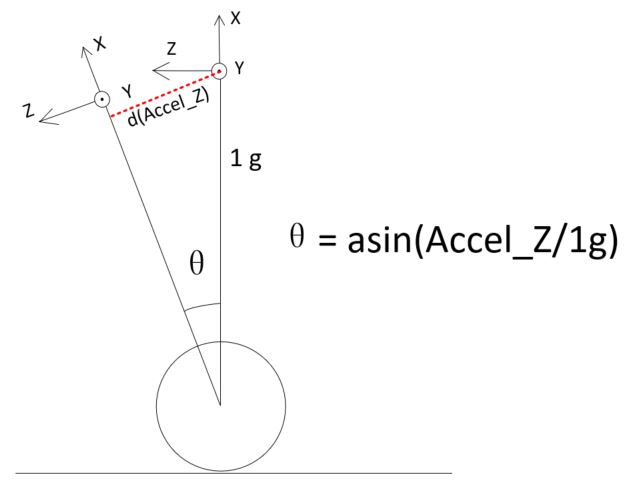If the car leans forward, the wheels should move forward to prevent it from tipping over. If it leans backward, wheels should move backward.
The vertical acceleration is always 1 g, so the angle can be calcualted using the asin() fuction: Theta = asin(Accel_Z/1g).
The result of this equation is in radians, to convert it to angles, you need to multiply it by 180/pi (or 360/2pi), which is 57.296: Theta = asin(Accel_Z/1g) x 57.296. To implement it in Arduino:

asin((float)accelerometer_data_raw/8192.0)* 57.296  // 8192 is the LSB/g configuration for the MPU chip

The Accel_Z value may have a little offset when you hold your 2-wheel balanced car up right. Mount the sensor to the car, test the Accel_Z offset and substract it from the the Accel_Z readings every cycle.
Mine Accel_Z offset is 450 so it was subtracted from the reading:

Declare it as follows:

int acc_calibration_value = -450;

and subtract it in the loop() function:

Wire.beginTransmission(MPU);                                              //Start communication with the gyro
Wire.write(0x3F);                                                         //Start reading at register 3F
Wire.endTransmission();                                                   //End the transmission
Wire.requestFrom(MPU, 2);                                                 //Request 2 bytes from the gyro
accelerometer_data_raw = Wire.read()<<8 | Wire.read();                      //Combine the two bytes to make one integer
accelerometer_data_raw += acc_calibration_value;                          //Add the accelerometer calibration value

For Gyro's readout data is angular velocity (degree/s), the loop time is 4000 us, so the Gyro raw data should be converted in degrees using the following formula:

Gyro Raw Data (delta angle change in LSB/degree/s) x 0.004 s /(131 LSB/degree/s) = Gyro Raw Data x 0.00031The angle accumulates. All new angles are results of displacement of the original angles. The way to get he current angle is to keep track all the previous angles but not just record the most recent 'delta_degree).
To implement it in Arduino:

gyro_pitch_data_raw -= gyro_pitch_calibration_value;                      //Subtract the gyro calibration value
angle_gyro += gyro_pitch_data_raw * 0.000031;                             //Calculate the traveled angle during this loop angle and add this to the angle_gyro variable

Now, the Data Fusion is a very common technique to filter out the high-frequency components and keep the low frequency components.

angle_gyro = angle_gyro * 0.9996 + angle_acc * 0.0004;                    //Correct the drift of the gyro angle with the accelerometer angle

The angle_gyro variable should store the real angle it has travelled.

We have two measurements of the angle from two different sources. The measurement from accelerometer gets affected by sudden horizontal movements and the measurement from gyroscope gradually drifts away from actual value. In other words, the accelerometer reading gets affected by short duration signals and the gyroscope reading by long duration signals. These readings are, in a way, complementary to each other. Combine them both using a Complementary Filter and we get a stable, accurate measurement of the angle. The complementary filter is essentially a high pass filter acting on the gyroscope and a low pass filter acting on the accelerometer to filter out the drift and noise from the measurement.

Task 8: Mount the MPU6050 sensor/breadboard on the top of the car. Gently lean the car from 0 degree to 90 degree and -90 degree. Check if you are receiving 90 or -90 in the serial monitor. Video demonstration required.

The end of this tutorial------------------------------------------------------------